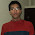## Friday, August 30, 2013

### Box Having Defective Balls. 10 Box 1 Chance.

You have been provided with 10 boxes. Each are full of balls and each ball weighs 10 gm.

One of these box has all defective balls. That is each ball in that box is of 9 gm.

You are also given a weighing machine. It is a digital electronic machine that shows weight of howsoever numebr of balls kept on it. That is if you put 'n' balls on it, it will tell you the total weight of n balls. And machine is big enough to accomodate any number of balls.

You have only one chance to weigh.

How will you find the box that is defective balls container.

1.Number the boxes from 1 to 10. Take as many number of ball from each box as the number present on the box.For example. Take 1 Ball from box number 1, 2 ball from box number 2...6 balls from box number 6 and so on upto box number 10. Now put all these ball from on weighing machine. Total sum should be 10+20+30+...+100=550 but we have one box with 9 gms balls..so we will get diff weight..so req box number is 550-(total weight of ball with defective balls).

2.There should be minimum 10 balls in each box for this to work.isnt it?

pavzie

3.Hi Sheetal Garg. Correct answer.
Great going Dude!!